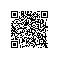# 平均要取多少个(0,1)中的随机数才能让和超过1

from __future__ import division
import random
N = 1000000
sums = 0
for i in range(N):
count = 0
s = 0
while 1:
s += random.random()
count += 1
if s > 1:
sums += count
break
print sums / N

 1 2.716957 2.718334  2.71885

2.718不就是e的味道吗，下面证明一下∫(0..1) (x^2)*1/2 dx = 1/6

∫(0..1) (x^3)*1/6 dx = 1/24

(1 – 1/n!) – (1 – 1/(n-1)!) = (n-1)/n!

∑(n=2..∞) n * (n-1)/n! = ∑(n=1..∞) n/n! = e使用钉钉扫一扫加入圈子
+ 订阅